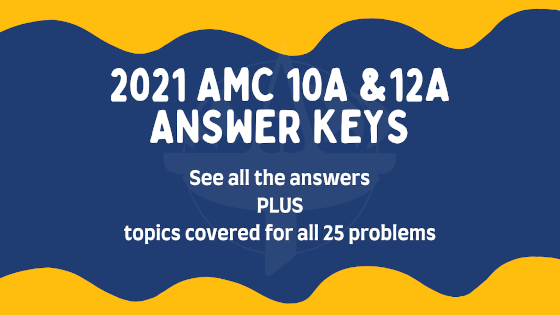# 2021 AMC 10A & AMC 12A Answer Key ReleasedPosted by

Yesterday, thousands of middle school and high school students participated in this year’s AMC 10A and 12A Competition. Hopefully everyone was able to take the exam safely, whether they took it online or in school!

The problems can now be discussed! See below for answer keys for both the 2021 AMC 10A and AMC 12A questions as well as the concepts tested on each problem.

1
D
arithmetic and exponents
2
C
algebra: ratios
3
D
number theory: place values and arithmetic
4
D
algebra: arithmetic sequences with motion
5
B
algebra: averages
6
A
algebra: motion
7
D
logic and cases
8
E
number theory: place values and repeating decimals
9
D
algebra: factoring and minimums
10
C
algebra: factoring and differences of squares
11
E
number theory: bases and divisibility
12
E
geometry: volumes and cones
13
C
geometry: volumes and pyramids
14
A
algebra: polynomials and Vieta’s theorem
15
C
algebra and combinatorics: quadratics and cases
16
C
algebra: arithmetic and medians
17
D
geometry: areas and trapezoids
18
E
number theory and algebra: prime factorizations and functions
19
E
algebra and geometry: absolute values, graphing, circles and areas
20
D
combinatorics: cases and logic
21
C
geometry: hexagons, equilateral triangles, and area
22
B
algebra and number theory: Diophantine equations
23
D
combinatorics: cases and total probability
24
D
geometry and algebra: graphing, areas, parallelograms
25
E
combinatorics: cases and symmetry

1
B
arithmetic and exponents
2
D
algebra and logic: square roots
3
D
number theory: place values and arithmetic
4
D
logic and cases
5
E
number theory: place values and repeating decimals
6
C
combinatorics: probability
7
D
algebra: factoring and minimums
8
C
number theory: sequences, parity, and patterns
9
C
algebra: factoring and differences of squares
10
E
geometry: volumes and cones
11
C
geometry: coordinates, reflections, and distance
12
A
algebra: polynomials and Vieta’s theorem
13
B
algebra: complex numbers and powers
14
E
algebra: logarithms, sums and summation notation, patterns
15
D
combinatorics: groups and cases
16
C
algebra: arithmetic and medians
17
D
geometry: areas and trapezoids
18
E
number theory and algebra: prime factorizations and functions
19
C
algebra: trigonometric functions
20
B
algebra and geometry: parabolas and distances
21
A
algebra and geometry: complex numbers, ellipses, and eccentricity
22
D
algebra: trigonometric identities and Vieta’s theorem
23
D
combinatorics: cases and total probability
24
D
geometry: circles, tangents, and triangles
25
E
number theory: prime factorizations and the divisor function

In total, 11 questions appeared on both the AMC 10B and AMC 12B. They are listed below:

Want full solutions to all the 2021 AMC 10A & 12A questions? Our full solutions review video has you covered! Click here for registration. The 2021 AMC 10A and 12A problems are available on the ZIML site along with previous exams. Click here for AMC 10 and here for AMC 12.

Starting to prep for the AIME? Join our Online AIME Two Day workshop on February 14th and 15th to prepare for next month’s AIME. Click here for more details and registration.

The AMC 10 and 12 exams are administered by the Mathematical Association of America (MAA). For general information about the AMC 10 and 12, click here.